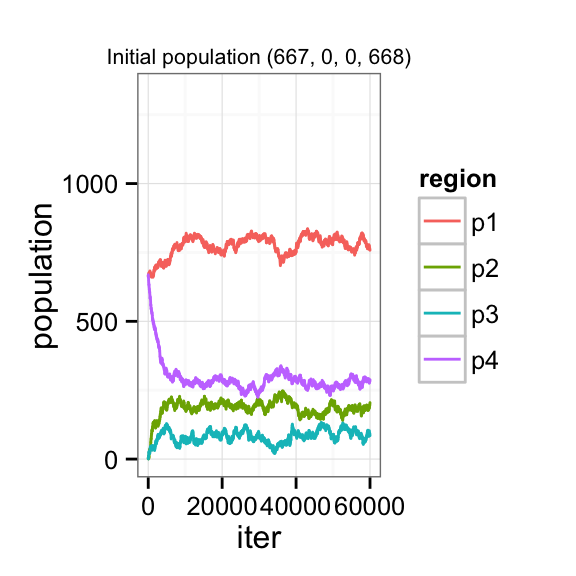In this paper, we build on Pan and Nagurney’s Markov Chain migration model and create a Markov Chain Monte Carlo model for migration. We continue to view migration as a chained phenomenon, represented by a random walk through possible population distributions rather than a deterministic markov chain. These simulations can show us the dynamics of reaching equilibrium, and the sensitivity of equilibrium and convergence to initial populations, costs, and utility.

## Introduction

Markov Chains have a long history of being used as theoretical models for studying human mobility. Pan and Nagurney (Pan, Nagurney 1994) provide a useful survey of past attempts at using Markov Chains, and advance the thinking by allowing for the possibility of non-homogenous markov chains. In their model and review of the literature, geographical locations are seen as states of the markov chain. Previous attempts at modeling population using markov chains involved a single transition matrix that could be calculated. Pan and Nagurney, by introducing costs, introduce the possibility of a nonhomogenous markov chain that still tends towards equilibrium.

Pan and Nagurney’s models can give insight into the dynamics of population mobility, but are limited by their deterministic nature. Costs can influence the time it takes to reach equilibrium and represent imperfect information or time lags associated with moving decisions in the real world, but once equilibrium is reached population is assumed to remain static. The authors point out that equilibrium does not necessarily mean there is no more migration, but simply that the probabilities of moving between locations have equalized, and net migration remains at zero.

In this paper we provide an alternative way of looking at a population model using markov chain monte carlo. By introducing randomness into the model, we hope to gain some insight into how exactly our population will converge to equilibrium, and what sort of variation will occur once equilibrium is acheived. In this way, we hope to model both migration induced by comparative utilities across regions, and movement due to random factors.

The choice of markov chain monte carlo led to some necessary departures from Pan and Nagurney’s model. Each iteration of Pan and Nagurney’s markov chain represents an equilibrium between comparative utilities and the cost of moving, and this equilibrium is reached by considering a flow of migrants. The iterations in our model do not represent equilibrium, but are rather defined steps towards an equilibrium population distribution. Each step represents the possibility of one person moving. Because of this, cost is not dependent on a flow of people, but is rather assumed to be a static, dependent only on the source and destination.

Pan and Nagurney look at network equilibrium models as one-step models. They arrive at equilibrium, but do not allow one to view chain migration nor the dynamics of migration. By using markov chain monte carlo, introducing randomness allows us another way to view these features of a migration model.

## Literature Review

Our model is based directly off of the Pan and Nagurney model, repurposed for use with markov chain monte carlo.

The authors define utility functions, $$u_i$$ for each location $$i$$, which are dependent on the populations of each region. Costs are a function of the flow between two locations, so each bilateral pair $$i$$ and $$j$$ has its own cost $$c_{ij}$$.

Starting from an initial population $$p_0$$, the flow between locations $$i$$ and $$j$$ can be discovered by solving the below equation:

$u_i (p^1_i) + c_{ij} (f_{ij}^1) = u_j (p^1_j)$

For each i and j.

The two region example shown in Pan and Nagurney’s paper assumed utility functions of $$u_1 (p) = -p_1 + 8$$ and $$u_2 (p) = -p_2 + 14$$. Costs were simply based on flow, so $$c_{12} (f_{12}) = 2f_{12}$$ and $$c_{21} (f_{21}) = 2f_{21}$$.

The solution to this toy model is explained and diagrammed below: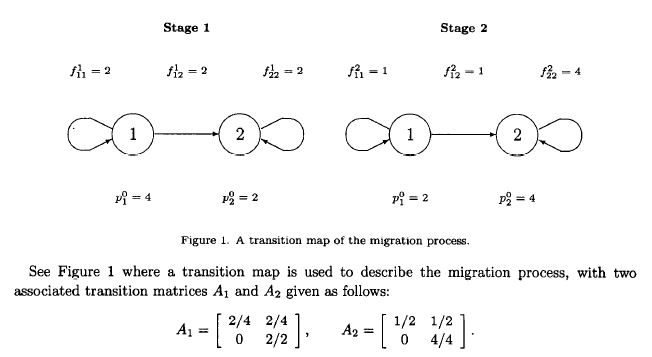In this case, the transition matrix is the same (and the derivation shows in what way they will differ.)

Pan and Nagurney reference an earlier paper written by Nagurney: A Network Model of Migration Equilibrium with Movement Cost (Nagurney 1990). In this paper, Nagurney provides a very similar model to population, but does not express it in terms of markov chains. Costs are assumed to be fixed, rather than based on flow. Utility functions are similar to the ones represented in the markov chain model, and are formally defined as $$u_i = -a_i p_i + b_i$$.

Nagurney shows that the question of optimal flows and equilibrium populations is solveable using quadratic programming. Given utility defined above, and costs defined as $$c_{ij}$$, the problem becomes:

Minimize

$\sum_i \frac{1}{2} a_i(\sum_{k \neq 1} f_{ki} - \sum_{k \neq 1} f_{ik} + \bar{p_i})^2 - \sum_{i} b_i (\sum_{k \neq 1} f_{ki} - \sum_{k \neq 1} f{ik} + \bar{p_i}) + \sum_{i,k \neq i} c_{ik} f_{ik}$

Subject to

$\sum_k f_{ik} \leq \bar{p_i}, \forall i$

and

$f_{ik} \geq 0, \forall i$

After defining the problem, Nagurney suggests an algorithm to solve it. Problems like this were solved step by step and numerically using markov chains when they were introduced to the model.

Other attempts at explaining migration using markov chains include A. Constant’s and K. Zimmerman’s paper “The Dynamics of Repeat Migration: A Markov Chain Analysis.” (Constant, Zimmerman 2012.) Using a Markov Chain approach to model repeat migration, this paper aims to describe the dynamics of repeated exit and entry between two countries. The paper estimates the transition probabilities using the formula $$P(E_{t+1} = i | E_t = j) = \frac{e^{\beta} ij^{x} mt}{\sum e^{\beta} ik^{x} mt}$$ using empirical data from Germany. These irepresent the coefficients on the personal characteristics variables for transition state i (note that only two transition state coefficient vectors are calculated as the other two are complements and calculated by taking 1 - P) and are estimated using logistic regression.

The paper then converts micro level longitudinal migration data at the individual level to person-years, which are treated as separate observations, to estimate these conditional probabilities. The end result are empirically-derived formulae that describe the personal characteristics that influence the decision to (repeat) migrate.

This approach is an interesting twist on the traditional Markov Chain analysis by detailing a particular case and integrating real world information about migrants. This could be useful for future considerations of our model as a guide to link real world data to our currently theoretical utility functions.

### Methodology

Our model takes utilities and costs and models an individual’s decision to move as a step in a markov chain monte carlo algorithm.

Considering $$n$$ locations, in its broadest terms the algorithm works as follows:

• Pick a source location, $$i$$, sampling from all locations with non-zero populations with equal weights.

• Pick a destination location, $$j$$, sampling from all locations (except for i) with equal weights (an individual may move to a location with a current population of zero.)

• Compare $$u_i (p) + c_{ij}$$ to $$u_j (p)$$

• If $$u_i (p) + c_{ij} \geq u_j (p)$$, accept the move with probability 1.

• If $$u_i (p) + c_{ij} < u_j (p)$$, accept the move with some probability.

The first major departure needed to adopt Pan and Nagurney’s model to MCMC is a subtle one. Given $$n$$ locations, Pan and Nagurney’s markov chain has $$n$$ states. In our model, each possible population distribution is a state of our markov chain, and we’re randomly walking around this distribution space. This state can be described as the total number of population vectors $$p = [p_1, p_2, p_3, ..., p_n]$$ with a total population $$t$$ such that $$\sum_{i=1}^n p = t$$.

The general form of the probability $$a$$ of accepting a state change to proposal state $$x'$$ drawn from probability distribution $$Q(p)$$ from state $$x_t$$ is:

$a = \frac{P(x')}{P(x_t)} \frac{Q(x_t | x')}{Q(x' | x_t)}$

$$\frac{P(x')}{P(x_t)}$$ is calculated by comparing the utility $$u_i (p)$$ of staying in location $$i$$ with the utility $$u_j (p) - c_{ij}$$ of moving. So:

$\frac{P(x')}{P(x_t)} = \frac{u_j (p) - c_{ij}}{u_i (p)}$

The second ratio is a test to see if the proposal distribution is symmetric. The proposal distribution is based on the distribution space defined above. By picking a random source and random destination, we are limiting ourselves to the number of states acheivable by moving one person from one location to another. Given $$n$$ locations, and $$n_{p \neq 0}$$ locations with non-zero populations, and the current popuation distribution state $$x_t$$, the proposal distribution $$Q(x' | x_t)$$ includes these $$n_{p \neq 0} (n-1)$$ states weighted equally, with zero probability of reaching any other state.

In most cases, our proposal distribution is symmetric. If we’re considering a move between locations $$i$$ and $$j$$, representing a change in state from $$x_t$$ to $$x'$$, and locations $$i$$ and $$j$$ are both non-zero, the number of states accessible from either $$x_t$$ or $$x'$$ is simply $$n_{p \neq 0} (n-1)$$. The probability $$Q(x_t | x') = Q(x' | x_t) = \frac{1}{n_{p \neq 0} (n-1)}$$.

The only state changes of $$x_t$$ to $$x'$$ that aren’t symmetric are $$x_t$$’s where the populations $$p_i = 1$$ or $$p_j = 0$$. In these cases, the number of locations with non-zero populations change, for obvious reasons, so $$Q(x_t | x') \neq Q(x' | x_t)$$. Interestingly, if $$p_i = 1$$ and $$p_j = 0$$, the situation remains symmetric.

This means our final probability $$a$$ of accepting a move from location $$i$$ to $$j$$, representing a state change from state $$x_t$$ to state $$x'$$ is:

$a = \frac{u_i (p)}{u_j (p) - c_{ij}} \frac{1/(n_{p \neq 0, x_t} (n-1))}{1/(n_{p \neq 0, x'} (n-1))}$

Our code can be found in Appendix A. Combining our general algorithm above with the theory, the probability with which we accept a move is calculated as $$a$$. If $$a$$ is greater than one, we accept the move. If $$a$$ is less than one, then $$a$$ represents the probability of accepting the move.

### Model dynamics

In this section, model dynamics will be examined. The first subsection will build a base model to use as a benchmark and examine what steady state populations these initial parameters generate. Subsequent subsections will examine various parameter perturbations against this baseline model in order to explore the dynamics of the model.

#### Base model

The base model relies upon arbitrarily chosen parameter values.

source('sensitivity_analysis.R')

params <- reset.params()
params$c # matrix of costs for moving from region i (row) to j (col) ## [,1] [,2] [,3] [,4] ## [1,] 0 200 400 600 ## [2,] 200 0 200 400 ## [3,] 400 200 0 200 ## [4,] 600 400 200 0 Initial utility is: params$k - params\$p
##  2021 1253 1426 1765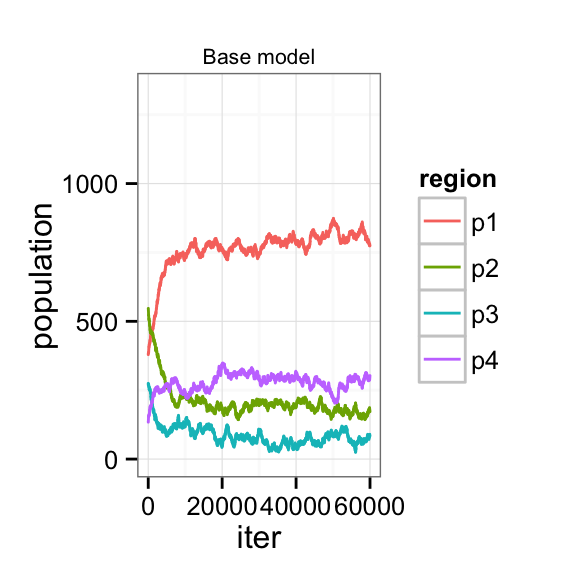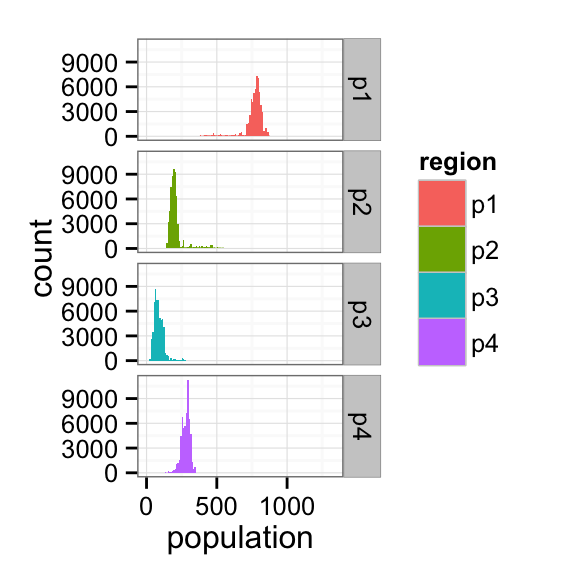##  region pop.mean ss.utility change.in.utility
##      p1 786.6773   1613.323         -407.6773
##      p2 189.2110   1610.789          357.7890
##      p3  76.0133   1623.987          197.9867
##      p4 283.0983   1616.902         -148.0983

We see that the populations converge after roughly 10,000 iterations, with relative stability across the different regions beyond that point. In the case of region 1, the population appears to converge to a steady state population of roughly 800. Region 2, 3, and 4 converge to equilibrium populations of roughly 200, 100, and 300, respectively. Steady state utilities across regions do not vary by much.

#### Initial conditions

Now that a base model has been run and examined, it is possible to explore whether its results are robust to changes in initial conditions. In the base model, the initial populations are 379, 547, 274, 135 for regions 1-4, respectively. Suppose the initial populations are instead, 0, 0, 0, 1335.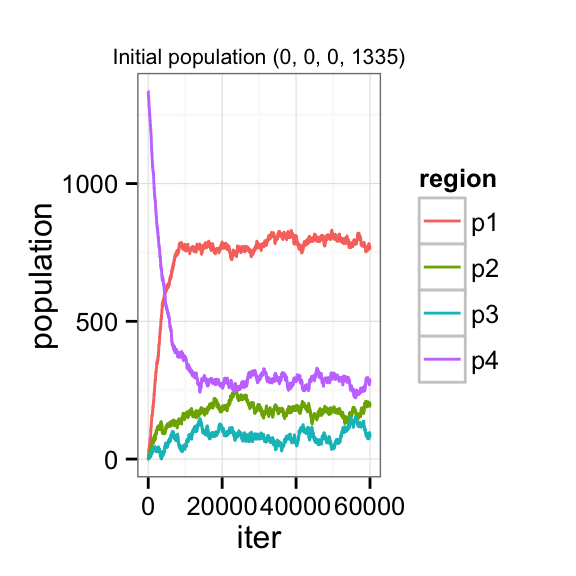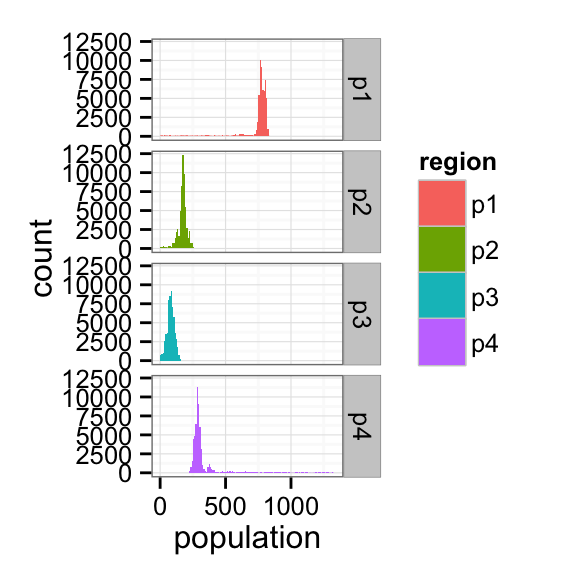##  region  pop.mean ss.utility change.in.utility
##      p1 781.88078   1618.119         -402.8808
##      p2 181.02868   1618.971          365.9713
##      p3  87.55692   1612.443          186.4431
##      p4 284.53362   1615.466         -149.5336

The steady state populations and utilities are remarkably similar to the base model.

Suppose the population is split evenly between regions 1 and 4.